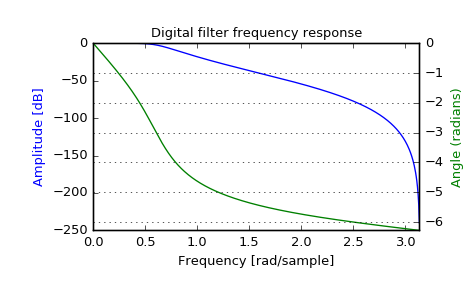# scipy.signal.freqz_zpk¶

scipy.signal.freqz_zpk(z, p, k, worN=None, whole=False)[source]

Compute the frequency response of a digital filter in ZPK form.

Given the Zeros, Poles and Gain of a digital filter, compute its frequency response:

:math:H(z)=k \prod_i (z - Z[i]) / \prod_j (z - P[j])


where $$k$$ is the gain, $$Z$$ are the zeros and $$P$$ are the poles.

Parameters: z : array_like Zeroes of a linear filter p : array_like Poles of a linear filter k : scalar Gain of a linear filter worN : {None, int, array_like}, optional If None (default), then compute at 512 frequencies equally spaced around the unit circle. If a single integer, then compute at that many frequencies. If an array_like, compute the response at the frequencies given (in radians/sample). whole : bool, optional Normally, frequencies are computed from 0 to the Nyquist frequency, pi radians/sample (upper-half of unit-circle). If whole is True, compute frequencies from 0 to 2*pi radians/sample. w : ndarray The normalized frequencies at which h was computed, in radians/sample. h : ndarray The frequency response.

freqs
Compute the frequency response of an analog filter in TF form
freqs_zpk
Compute the frequency response of an analog filter in ZPK form
freqz
Compute the frequency response of a digital filter in TF form

Notes

Examples

>>> from scipy import signal
>>> z, p, k = signal.butter(4, 0.2, output='zpk')
>>> w, h = signal.freqz_zpk(z, p, k)

>>> import matplotlib.pyplot as plt
>>> fig = plt.figure()
>>> plt.title('Digital filter frequency response')

>>> plt.plot(w, 20 * np.log10(abs(h)), 'b')
>>> plt.ylabel('Amplitude [dB]', color='b')

>>> ax2 = ax1.twinx()
>>> angles = np.unwrap(np.angle(h))
>>> plt.plot(w, angles, 'g')